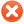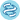Plot options# Use multiple genesUse list of genes Use mean expression of selected genes Use ratio of two genes Filter by median expression
more genes param

# ExampleExample input for multiple genes:
1007_s_at 1
200000_s_at -1
31637_s_at 0.5
40016_g_at -2

Explanation:
first: normal,
second: gene is inverted,
third: gene has weight,
fourth: gene has weight and is inverted

You can also use following format:
1007_s_at, 1
200000_s_at, -1
31637_s_at, 0.5
40016_g_at, -2

 Split patients by --none--lower quartilelower tertilemedianupper tertileupper quartile Auto select best cutoffTrichotomization : --none--T1 vs T3Q1 vs Q4Survival: OS (n=7462)RFS (n=4420)Compute median survival:Follow up threshold: all2 months4 months6 months12 months18 months24 months30 months36 months48 months60 months120 months180 months240 monthsCensore at threshold:Select all (n=7489) Bladder Carcinoma (n=405) Breast cancer (n=1090) Cervical squamous cell carcinoma (n=304) Esophageal Adenocarcinoma (n=80) Esophageal Squamous Cell Carcinoma (n=81) Head-neck squamous cell carcinoma (n=500) Kidney renal clear cell carcinoma (n=530) Kidney renal papillary cell carcinoma (n=288) Liver hepatocellular carcinoma (n=371) Lung adenocarcinoma (n=513) Lung squamous cell carcinoma (n=501) Ovarian cancer (n=374) Pancreatic ductal adenocarcinoma (n=177) Pheochromocytoma and Paraganglioma (n=178) Rectum adenocarcinoma (n=165) Sarcoma (n=259) Stomach adenocarcinoma (n=375) Testicular Germ Cell Tumor (n=134) Thymoma (n=119) Thyroid carcinoma (n=502) Uterine corpus endometrial carcinoma (n=543)

## Restrict analysis to subtypes...

 Stage: all 1 (n=1790) 2 (n=1707) 3 (n=1269) 4 (n=676) Gender: all female (n=4305) male (n=3184) Race: all white (n=5485) asian (n=557) black/african american (n=733) Grade: all 1 (n=304) 2 (n=1297) 3 (n=1510) 4 (n=93) Mutation burden: all high (n=3510) low (n=3390) Neoantigen load: all high (n=3386) low (n=3012)

## Restrict analysis based on cellular content…

 Basophils: all enriched (n=3319) decreased (n=3698) B-cells: all enriched (n=3763) decreased (n=3254) CD4+ memory T-cells: all enriched (n=3791) decreased (n=3226) CD8+ T-cells: all enriched (n=3601) decreased (n=3416) Eosinophils: all enriched (n=3160) decreased (n=3857) Macrophages: all enriched (n=3983) decreased (n=3034) Mesenchymal stem cells: all enriched (n=3508) decreased (n=3509) Natural killer T-cells: all enriched (n=3165) decreased (n=3852) Regulatory T-cells: all enriched (n=3434) decreased (n=3583) Type 1 T-helper cells: all enriched (n=3334) decreased (n=3683) Type 2 T-helper cells: all enriched (n=3942) decreased (n=3075)

Please note: the generated p value does not include correction for multiple hypothesis testing by default.n = number of patients with available clinical data

Please kindly cite following paper to support further development: Nagy A, Munkacsy G, Gyorffy B: Pancancer survival analysis of cancer hallmark genes, Sci Rep., 2021 Mar 15;11(1):6047, https://doi.org/10.1038/s41598-021-84787-5